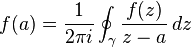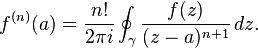##Theorem

Suppose U is an open subset of the complex plane C, f : UC is a holomorphic function and the closed disk D = { z : | zz0| ≤ r} is completely contained in U. Letbe the circle forming the boundary of D. Then for every a in the interior of D:where the contour integral is taken counter-clockwise.

The proof of this statement uses the Cauchy integral theorem and similarly only requires f to be complex differentiable. Since the reciprocal of the denominator of the integrand in Cauchy’s integral formula can be expanded as a power series in the variable (a − z0), it follows that holomorphic functions are analytic. In particular f is actually infinitely differentiable, withThis formula is sometimes referred to as Cauchy’s differentiation formula.

The circle γ can be replaced by any closed rectifiable curve in U which has winding number one about a. Moreover, as for the Cauchy integral theorem, it is sufficient to require that f be holomorphic in the open region enclosed by the path and continuous on its closure.

This proof is based on the fact that the value of the integral is invariant under deformation as long as the integrand is analytic over this closed area.

Annonser## Om mattelararen

Licentiate of Philosophy in atomic Physics Master of Science in Physics
Det här inlägget postades i Calculus, Imaginary numbers och har märkts med etiketterna . Bokmärk permalänken.